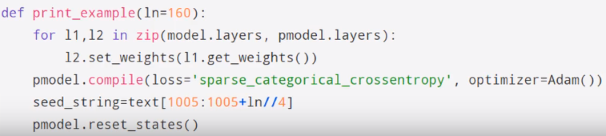# Predicting with the stateful RNN in lesson 6

Hi,

I was hoping for a hint about prediction with the improved stateful RNN, with multiple LSTM layers, that is seen in the video for lesson 6, around the 1h20 mark. The lesson6.ipynb notebook doesn’t illustrate this and the helper `print_example()` function given in the char-rnn.ipynb notebook for the non-stateful RNN doesn’t work. In the latter case, the problem is that the dimensionality of the layers is different. Here is the final one, as reported by Keras:

`timedistributed_23 (TimeDistribu (512, 64, 83)`

Prediction therefore requires a (512, 64) input, but `print_example()` generates a (1, 64) input per step. In the video, there is a glimpse of part of an alternate `print_example()`:This is generating some kind of supplementary model I think, called `pmodel`. I presume this is adding some additional layer (or multiple) on the end to facilitate prediction, but I’m not sure what to try. Does anyone have any suggestions please? Or is that other char-rnn-bn.ipynb notebook available somewhere that I have missed?

I have (sort of) managed to get it to work, by padding the (1, 64) input and pulling out a single row from the predictions, but this doesn’t feel like quite the right solution!

Thanks.

Below is one solution, following on from the snippet in the video. I think it’s correct, but better suggestions very welcome!

(Some of the variable names are slightly different from the original notebooks, but hopefully obvious enough.)

``````def make_model(batch_size_override=None):
if batch_size_override is None:
batch_size_override = batch_size
model = Sequential([
Embedding(input_dim=vocab_size, output_dim=num_latent_factors,
input_length=max_sequence_len,
batch_input_shape=(batch_size_override, max_sequence_len)),
# ...
])
return model

def print_example(seed_string, gen_length=320):
pred_model = make_model(batch_size_override=1) # This is the important bit
for layer, pred_layer in zip(model.layers, pred_model.layers):
pred_layer.set_weights(layer.get_weights())

output = seed_string
for i in range(gen_length):
text_fragment = [char_to_idx[c] for c in output[-max_sequence_len:]]
predict_batch = np.array(text_fragment)[np.newaxis,:]
prediction = pred_model.predict(predict_batch, verbose=0, batch_size=1)[-1]
prediction = prediction / np.sum(prediction)
output += np.random.choice(distinct_chars, p=prediction)
print(output)
return output
``````